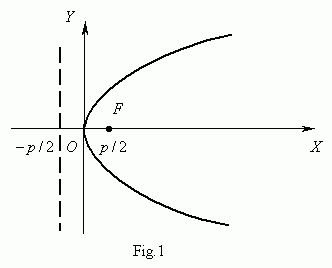Parabola

Parabola. Focus. Directrix. Equation of parabola.

Equation of tangent line to parabola.

Tangency condition of straight line and parabola.

A parabola ( Fig.1 ) is called a locus of points, equidistant from the given point F , called a focus of parabola, and the given straight line, not going through this point

and called a directrix of parabola.An equation of parabola ( Fig.1 ) is :

y 2 = 2 p x .

The axis ну is here an axis of symmetry of parabola.

Let п ( У 1 , С 1 )  be a point of parabola, then an equation of tangent line to parabola in this point is :

С 1 y = p ( x + У 1 ) .

A tangency condition of a straight line y = m x + k and a parabola y 2 = 2 p x :

2 m k = p .